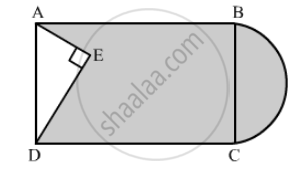Advertisement Remove all ads

# In the Following Figure, from a Rectangular Region Abcd with Ab = 20 Cm, a Right Triangle Aed with Ae = 9 Cm and De = 12 Cm, is Cut Off. on the Other End, Taking Bc as Diameter, a Semicircle is Adde - Mathematics

Sum

In the following figure, from a rectangular region ABCD with AB = 20 cm, a right triangle AED with AE = 9 cm and DE = 12 cm, is cut off. On the other end, taking BC as diameter, a semicircle is added on outside' the region. Find the area of the shaded region. [Use π =]22/7 [CBSE 2014]Advertisement Remove all ads

#### Solution

In right triangle AED
AD2 = AE2 + DE2
= (9)2 + (12)2
= 81 + 144
= 225
∴ AD2 = 225
⇒ AD = 15 cm
We know that the opposite sides of a rectangle are equal
AD = BC =  15 cm
Area of the shaded region = Area of rectangle − Area of triangle  AED + Area of semicircle

$= AB \times BC - \frac{1}{2} \times AE \times DE + \frac{1}{2}\pi \left( \frac{BC}{2} \right)^2$

$= 20 \times 15 - \frac{1}{2} \times 9 \times 12 + \frac{1}{2} \times \frac{22}{7} \times \left( \frac{15}{2} \right)^2$

$= 300 - 54 + 88 . 3928$

$= 334 . 3928 {cm}^2$

Hence, the area of shaded region is 334.39 cm2

Is there an error in this question or solution?
Advertisement Remove all ads

#### APPEARS IN

RD Sharma Class 10 Maths
Chapter 13 Areas Related to Circles
Exercise 13.4 | Q 13 | Page 57
Advertisement Remove all ads

#### Video TutorialsVIEW ALL 

Advertisement Remove all ads
Share
Notifications

View all notifications

Forgot password?
Course